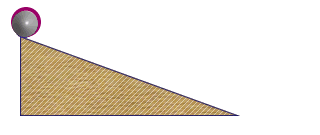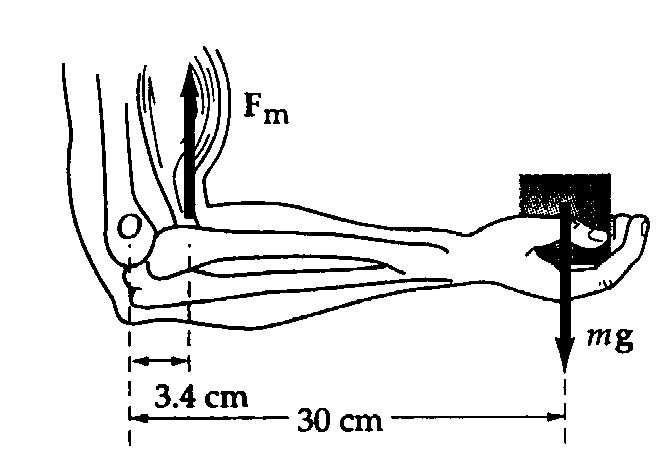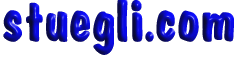# Rotational MechanicsNOTE: OUTLINE BELOW IS EXPANDABLE. CLICK ON HEADINGS TO SEE ADDiTIONAL INFORMATION

## PhyzxSpeek

• ### TORQUE

Torque is the product of the length of the lever arm and the component of the force perpendicular to the lever arm.

• ### LEVER ARM

The distance from the turning axis and the to the point where the force applied is called the LEVER ARM.

• ### ROTATIONAL INERTIA

Rotational Inertia is the resistance of an object to changes in its rotational state of motion. The terms moment of inertia   or  first moment are more commonly used to describe this property of an object or system.

• ### ROTATIONAL VELOCITY

The number of revolutions per unit time of a rotating or revolving object is know as the Rotational Velocity. This quantity is also referred to as Angular Velocity or Rotational Speed. The correct terminology is Angular Velocity as this is a VECTOR measure.

• ### ANGULAR MOMENTUM

Angular momentum is a property of an object or system that describes its resistance to changes in its rotational speed. It is quantified by the product of the Rotational Inertia and the Rotational Velocity.

• ### LAW OF CONSERVATION OF ANGULAR MOMENTUM

If no unbalanced external Torque acts on a rotating system, the angular momentum of that system is constant.

## TORQUE (click to expand)

• Torque = (force perpendicular to the lever arm) x (length of lever arm)

• Units

Newton-meters (metric) or Pound-feet (English)

• BALANCED TORQUES

When balanced torques act on an object, there is no change in rotation.

• TORQUE & CG

When the base of support is not under the center of gravity, the gravitational force produces a torque that causes toppling.

• INTERACTIVE SIMULATIONS

• Reel Whirled - Your Body## ROTATIONAL INERTIA (or moment of inertia) (click to expand)

• The resistance of an object to changes in its rotational state of motion is called rotational inertia.

The greater the rotational inertia, the harder it is to change the rotational speed of an object.

Different Axes

• Longitudinal

• Transverse

• Median

## ANGULAR MOMENTUM (click to expand)

• Angular momentum, the “inertia of rotation,” is the product of rotational inertia and rotational velocity.

Angular momentum is a property of an object or system that describes its resistance to changes in its rotational speed. It is quantified by the product of the Rotational Inertia and the Rotational Velocity.

Angular Momentum = = Rotational Inertia x Angular Velocity = I  x ω = mvr

## 'CONSERVATION OF' LAW (click to expand)

• Angular momentum is conserved when no external torque acts on a body.Copyright © 2005 -  S. B. EglI